# High School Math : Understanding Radicals

## Example Questions

2 Next →

### Example Question #1 : Expressing Radicals As Exponents

Simplify: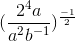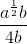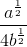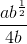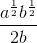Explanation:

Multiply the numerator and denominator to the exponent:Simplify the expression by combining like terms: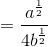### Example Question #11 : Understanding Radicals

Express the following exponent in radical form: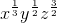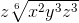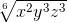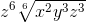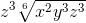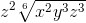Explanation:

Begin by converting each exponent to have a denominator of: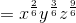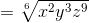Finally, simplify: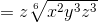### Example Question #61 : Algebra Ii

Express the following exponent in radical form: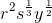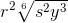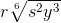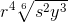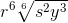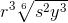Explanation:

Begin by converting each exponent to have a denominator of:Now, put this in radical form: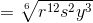Finally, simplify: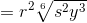### Example Question #62 : Algebra Ii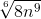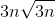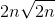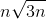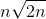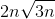Explanation:

Begin by converting the radical into exponent form: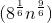Simplify the exponent and multiply: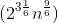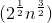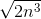Simplify:### Example Question #63 : Algebra Ii

Express the following exponent in radical form: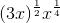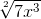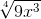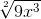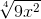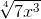Explanation:

Begin by changing the fractional exponents so that they both have a common denominator of: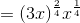Now, put this in radical form and simplify: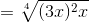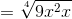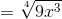2 Next →